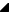﻿ TRSPieChart.Values Property# TRSPieChart.Values Property

Contains all the pie slices in the pie chart. Each pie slice ( RSCharts.TRSPieChartValue ) may have its own Color and Caption. The size of the pie slice is dependent on the Value, e.g., how much that value is proportional to the sum of all pie slice values in the pie chart. Use the Values collection to add, delete, and modify pie slices in the chart.

Namespace: RSChartsSyntax
 Delphi published   property Values: TRSPieChartValues read GetValues write SetValues stored IsValuesStored;

Property Value

Type: TRSPieChartValuesExamples
 The following code adds 3 pie slices to the chart: Delphi var    Value: TRSPieChartValue;  // note, this type should be the same that the chart uses (e.g., TRS2DChartValue, TRSArrowChartValue, etc)  begin    // Add first point    Value := RSPieChart1.Values.Add;    Value.Color := clRed;    Value.Value := 10.0;    // Add second point, twice as large as first    Value := RSPieChart1.Values.Add;    Value.Color := clGreen;    Value.Value := 20.0;    // Add third point, twice as large as second    Value := RSPieChart1.Values.Add;    Value.Color := clNavy;    Value.Value := 40.0;      // Draw the pie slices starting at 12:00 and go counter-clockwise    RSPieChart1.Offset := 90;  end;See Also

#### Reference

 • RSCharts.TRSPieChartValue
 • RSCharts.TRSPieChartValues
 • TRSPieChart
 • RSCharts

RiverSoftAVG Charting Component Suite (RCCS) © 2005-2015, Thomas G. Grubb